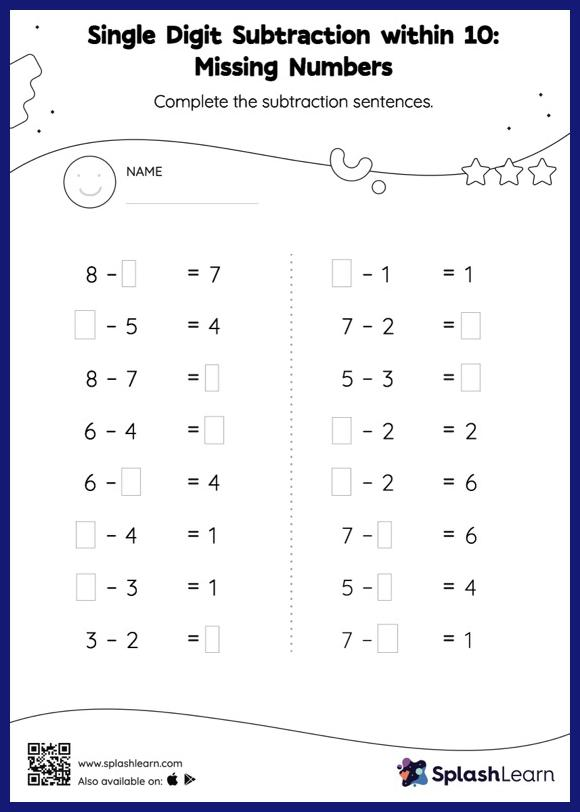# Single Digit Subtraction within 10: Missing Numbers Worksheet

Home > Single Digit Subtraction within 10: Missing NumbersIf a minuend or subtrahend is missing, students use the count-back method or the relationship between addition and subtraction to determine the missing number. Also, they subtract the numbers if the missing number is the difference of the subtraction sentence. This single digit subtraction within 10 worksheet offers a tonne of practice with this idea. In this worksheet, students practice solving problems written in the horizontal format. How numbers are laid out in a problem affects the method a student employs to solve it. Therefore to develop actual fluency and mastery of multiple strategies, students must practice different formats.